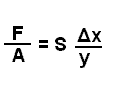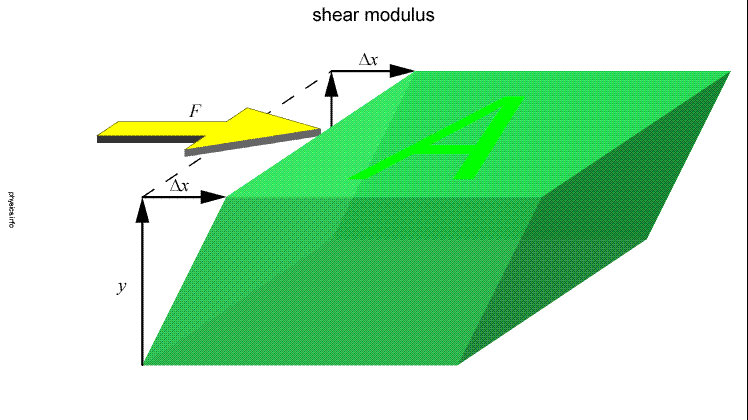# Shear stress and pressure drop

hello all... Please can i know physically why the pressure drop in a fluid when there's shear stress? what happens? thank you

davenn
Gold Member
Greetings Tonyik

Firstly
you cannot have shear stress in a fluid .... That is, fluids cannot support shear stress

This is how we found out that the outer core of the earth is liquid, because earthquake shear waves will not propagate through that region

knowing that, would you like to redefine your question and maybe give an example relating to your question

cheers
Dave

Chestermiller
Mentor
Greetings Tonyik

Firstly
you cannot have shear stress in a fluid .... That is, fluids cannot support shear stress

This is how we found out that the outer core of the earth is liquid, because earthquake shear waves will not propagate through that region

knowing that, would you like to redefine your question and maybe give an example relating to your question

cheers
Dave

Of course you can have shear stress in a fluid. According to Newton's law of viscosity, for a fluid that is being sheared between parallel plates, the shear stress is equal to the viscosity times the shear rate, with the shear rate equal to the relative velocity divided by the distance between the plates.

In a fluid flowing through a pipe, there is a viscous shear stress at the wall that acts in the direction opposite to the direction of fluid motion. This integrates to a tangential force at the wall. In order to overcome this force, you need a higher pressure at the inlet of the pipe than at the exit. For pipe flow, the pressure drop in the pipe is equal to 4L/D times the shear stress at the wall, where L is the length of the pipe and D is the diameter. The shear stress at the wall for laminar flow is equal to 8V/D times the viscosity, where V is the volumetric average velocity of the fluid.

Chet

davenn
Gold Member
Of course you can have shear stress in a fluid. According to Newton's law of viscosity, for a fluid that is being sheared between parallel plates, the shear stress is equal to the viscosity times the shear rate, with the shear rate equal to the relative velocity divided by the distance between the plates.

In a fluid flowing through a pipe, there is a viscous shear stress at the wall that acts in the direction opposite to the direction of fluid motion. This integrates to a tangential force at the wall. In order to overcome this force, you need a higher pressure at the inlet of the pipe than at the exit. For pipe flow, the pressure drop in the pipe is equal to 4L/D times the shear stress at the wall, where L is the length of the pipe and D is the diameter. The shear stress at the wall for laminar flow is equal to 8V/D times the viscosity, where V is the volumetric average velocity of the fluid.

Chet

my geophysics lecturer at university would have severely debated that with you
and would have give you the inverted jug of beer experiment to prove otherwise

if a fluid could support shearing then why do shear waves not travel through it ?

it just doesnt happen, Shear modulus of a fluid = 0

shear modulus
Shear Modulus (S) also known as the rigidity modulusGases and liquids can not have shear moduli. They have viscosity instead.

http://physics.info/elasticity/

Dave

#### Attachments

Last edited:
Chestermiller
Mentor
my geophysics lecturer at university would have severely debated that with you
and would have give you the inverted jug of beer experiment to prove otherwise

if a fluid could support shearing then why do shear waves not travel through it ?

it just doesnt happen, Shear modulus of a fluid = 0

shear modulus
Shear Modulus (S) also known as the rigidity modulusGases and liquids can not have shear moduli. They have viscosity instead.

http://physics.info/elasticity/

Dave
Who said anything about gasses and liquids supporting shear strain? You do know the difference between stress and strain, correct? The OP was asking about shear stresses in a liquid, and how they contribute to pressure drop. Either your lecturer didn't know much about fluid mechanics, or you misinterpreted what he was saying. Viscosity is precisely what we are talking about here. This is the fluid property that allows a fluid to develop shear stress. I hope you really didn't think that shear stresses could only exist in solids. In a liquid, the shear stress is proportional to the rate of strain, rather than the strain itself.

Chet

Yea... viscous fluid shear is a pretty fundamental part of fluid dynamics and boundary layer theory.

hello again... yes im talking of the shear stress due to the viscosity of the fluid..i dont know but if you google it you will get thousands of links about it .. and yes im not an expert in fluid mechanics and still i didnt know why Physically there's drop of pressure i know its to overcome the energy loss due to friction ect quotation from the book Fundamentals of Fluid Mechanics by Bruce Munson :
"The need for the pressure drop can be viewed from two different standpoints. In terms of a force balance, the pressure force is needed to overcome the viscous forces generated. In terms of an energy balance, the work done by the pressure force is needed to overcome the viscous dis- sipation of energy throughout the fluid"
i mean why the viscous forces causes pressure drop in a molecular view that's what i mean by physically... thanks again

Chet

thanks a lot i think i will like it

ahh okk i think i understand it if we want to make a flow in a pipe we have to make a pressure difference ( drop of pressure between the entrance and the exit) so when fluid is flowing there's the viscosity of the fluid causing the viscous forces... but why we say an ideal fluid doesnt have viscosity so it has no pressure drop in a pipe? so how an ideal fluid can flow without pressure drop?

Chestermiller
Mentor
There's no such thing as an ideal fluid. It is only an approximation to the behavior of a real fluid in the limit of vanishingly small viscosity. As you make the viscosity of a real fluid lower and lower, the pressure drop in the pipe gets lower and lower. Thus, for the same axial velocity, water will have a lower pressure drop than molasses.

As to the question of what is happening on the molecular scale that gives rise to viscosity, see Transport Phenomena.

Chet

sorry for re-openning this thread but really im not getting it look what i found:
But in fluid flow, DP is used to designate pressure drop, and thus it is P1 - P2
. A pressure drop due to viscous
effects represents an irreversible pressure loss, and it is called pressure loss
DPL to emphasize that it is a loss (just like the head loss hL

Note from Eq. 8–20 that the pressure drop is proportional to the viscosity
u of the fluid, and DP would be zero if there were no friction. Therefore,
the drop of pressure from P1 to P2 in this case is due entirely to viscous
effects

Im confused about why they call it a pressure loss? if it's necessary for a pressure drop in a fluid to flow in a pipe than why they called it pessure loss? and why they say if there's no friction there's no pressure drop? so how can an ideal fluid even in theory flow without a pressure drop... thanks again

http://www.uio.no/studier/emner/matnat/math/MEK4450/h11/undervisningsmateriale/modul-5/Pipeflow_intro.pdf even all the books they say its a pressure loss..

It is necessary for a pressure differential (i.e. the pressure at the inlet of the pipe is greater than the pressure at the outlet) for a fluid to flow in a pipe, not a pressure loss. Though all real fluids will have viscosity, and therefore some degree of shear, which results in pressure losses.

Last edited:
Chestermiller
Mentor
sorry for re-openning this thread but really im not getting it look what i found:

Im confused about why they call it a pressure loss? if it's necessary for a pressure drop in a fluid to flow in a pipe than why they called it pessure loss? and why they say if there's no friction there's no pressure drop? so how can an ideal fluid even in theory flow without a pressure drop... thanks again

http://www.uio.no/studier/emner/matnat/math/MEK4450/h11/undervisningsmateriale/modul-5/Pipeflow_intro.pdf even all the books they say its a pressure loss..

Some people call pressure drop "pressure loss" because, if the pressure at the inlet is higher than the pressure at the outlet, there is a "pressure loss." When they talk about "friction", what they really mean, more precisely, is viscous resistance.

When you ask the question "how can an ideal fluid even in theory flow without a pressure drop," this is analogous to asking "how can electric current pass through a zero resistance wire without a voltage difference across it." Even the smallest voltage difference across the wire will result in a huge current. For a finite current, the voltage difference would approach zero. Even the smallest pressure drop across the pipe will result in a huge flow. For a finite fluid flow, the pressure drop would approach zero. We are talking about the limit as the resistance approaches zero or as the fluid viscosity becomes vanishingly small.

•mraj